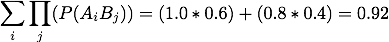You do not have java enabled for this browser.

# How To Use

### This is a signed Java Applet, the certificate must be accepted for the program to work.

At any point, the "Clear Display" button can be used to clear the large text box.
At any point, you can restart the process using a different transaction file.

1. Select the desired transaction file.
• Any non-zero value will be treated as a boolean true (or 1)
• A transaction file is required
2. Select/create the desired itemset output file
• This file is used when probability measures are generated and uses the following format:
• Line 1: Number of transaction
• Line 2: Number of items per transaction
• Line 3: ******
• Line 4+: [Itemset],[frequency]
• Lines containing '-' indicate an increase in the number of items in the itemset
• An output file is required
3. Enter a minSup (minimum support) value
• minSup indicates the minimum frequency that the candidate must have in order to be frequent
• This value reqresents a percentage and accepts decimals
• Correct: "60.1" to represent 60.1%
• Correct: "40" to represent 40%
• Incorrect: "0.4" to represent 40%
• A minSup is required. The value is originally set at 50.0
4. Enter the transaction file's item separator
• If it is space separated, ensure to enter ' ' into the field
• The item separator is required. The value is original set as ','
5. Click the button for the desired algorithm (Apriori or DIC) to calculate the frequent itemsets
6. Select/create the desired probability output file
• A probability output file is required
7. Select the probability measures from the list that you wish to apply to the frequent itemsets
• Selecting none is an option, as long as you enter a User Define Measure
• If you select "Interestingness Weighting Dependency", you must use the boxes above the list to specify a 'm' and 'k' value
8. If desired, enter a User Defined Measure
 Operation Graphical Representation User Defined Measure Example Probability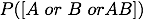P([A or B or AB]) P(A)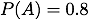logical not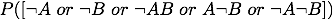P([~A or ~B or ~AB or A~B or ~A~B]) P(~A)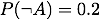Conditional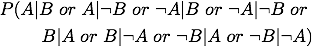P([A|B or A|~B or ~A|B or ~A|~B or B|A or B|~A or ~B|A or ~B|~A]) P(B|A)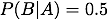Log base n (logn)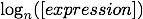\log{n, [expression]} \log{2, P(A)}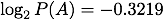Square Root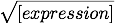\sqrt{[expression]} \sqrt{P(A)}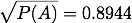Root n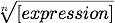\rt{n, [expression]} \rt{3, P(A)}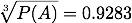Summation (Sigma)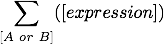\sigma{[A or B], [expression]} \sigma{B, P(AB)}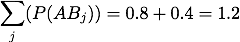Summation (Sigma) - AB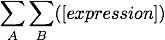\sigma{AB, [expression]} \sigma{AB, P(AB)}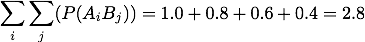Product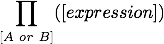\prod{[A or B], [expression]} \prod{B, P(AB)}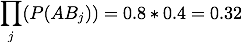Product - AB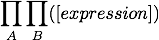\prod{AB, [expression]} \prod{AB, P(AB)}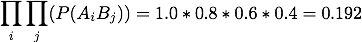Max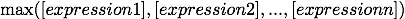\max{[expression 1], [expression 2], ... , [expression n]} \max{P(A),P(B),P(AB)}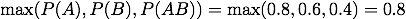Max[A or B]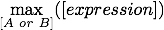\max{[A or B], [expression]} \max{A, P(AB)}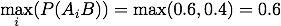MaxAB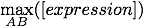\max{AB, [expression]} \max{AB, P(AB)}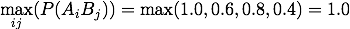Max nested in a Sigma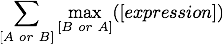\maxSigma{[A or B], [expression]} \maxSigma{A, P(AB)}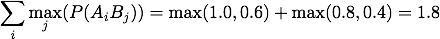Max nested in a Prod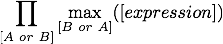\maxProd{[A or B], [expression]} \maxProd{A, P(AB)}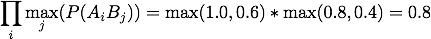Sigma nested in a Prod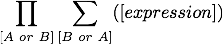\sigmaProd{[A or B], [expression]} \sigmaProd{A, P(AB)}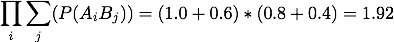Prod nested in a Sigma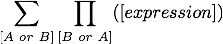\prodSigma{[A or B], [expression]} \prodsigma{A, P(AB)}